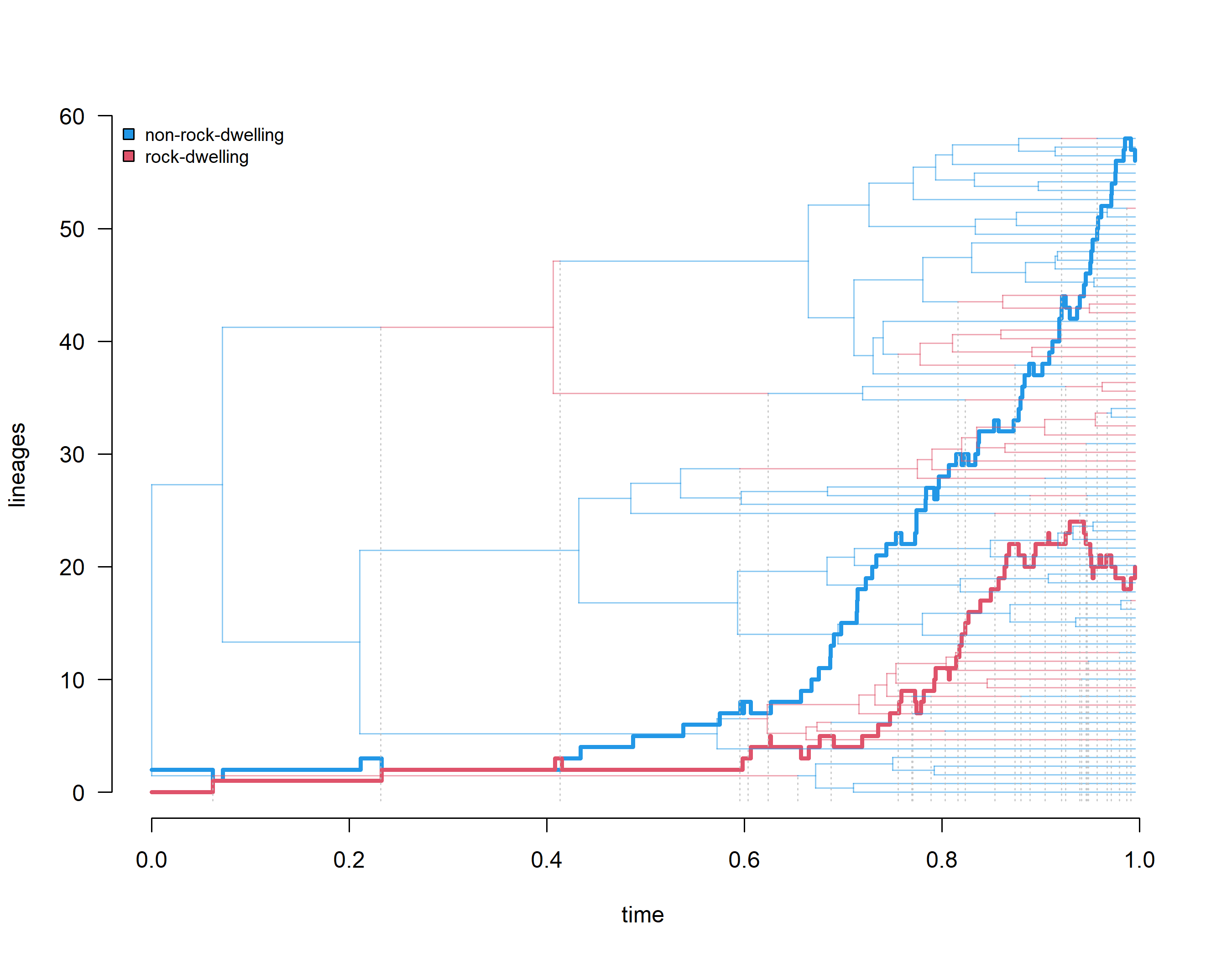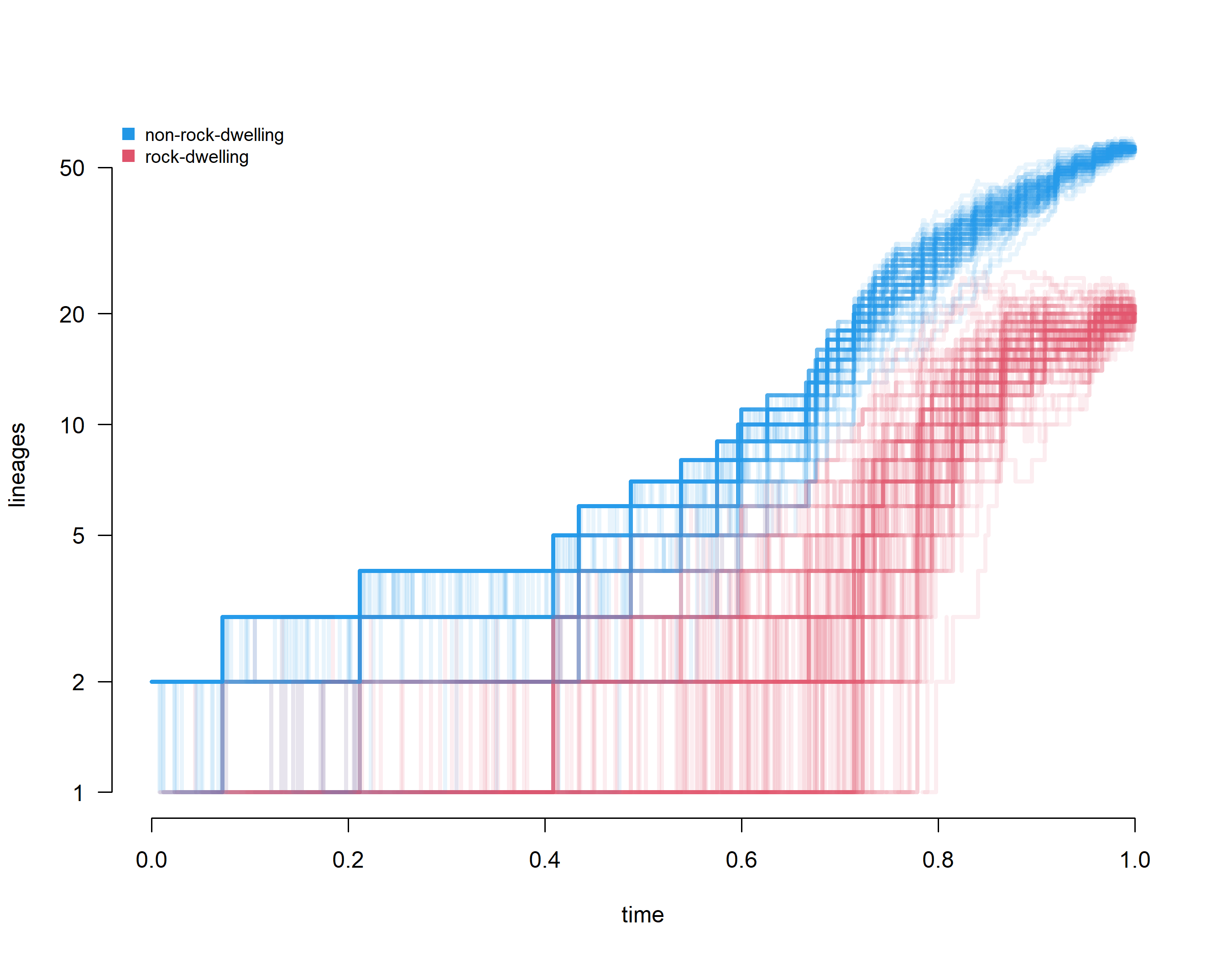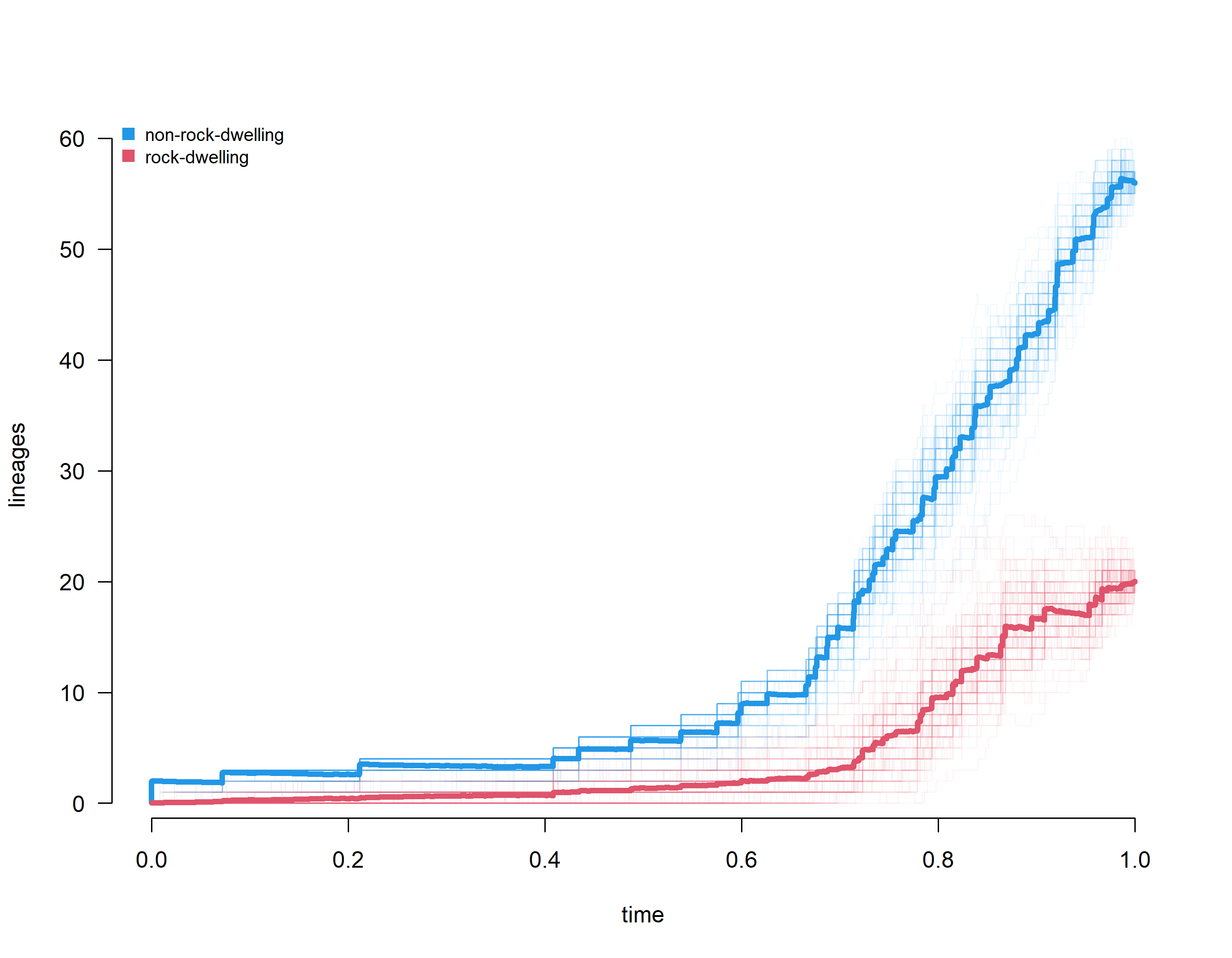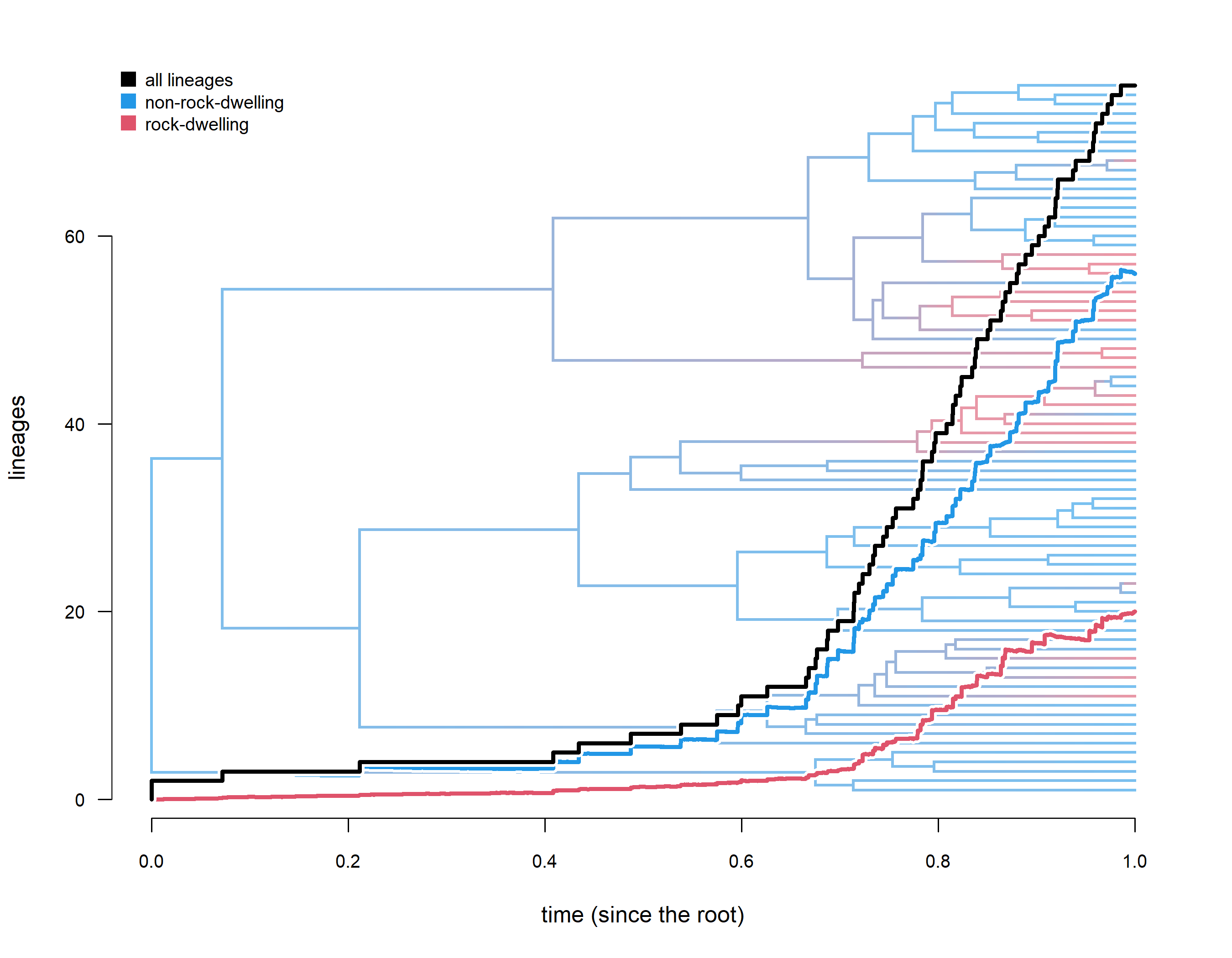## Saturday, July 30, 2022

### Creating a lineage through time plot showing the number of lineages in each state from stochastic mapping

A phytools user recently contacted me with the following interesting question:

“I was curious if there was any way to generate something somewhat similar to a 'lineage through time' plot, but for the branches of a stochastically mapped character (e.g. showing accumulation of new branches in a given state)? Or, alternatively, if there was a way to view the proportion of branches in a given character state through time? I’ve tried playing around with timeslice'ing simmaps and am a bit unsure how best to do this.”

Actually, I'm kind of surprised I've never shown how to do this before. (Or perhaps I have, and just forgotten.)

Also surprisingly, it's not all that difficult. I can think of a couple of ways to do this, and I'm going to demo the easiest.

For this example, I'll use a phylogeny & dataset for rock- and non-rock-dwelling tropidurid lizards that features in an upcoming paper by Ken Toyama, Luke Mahler, & I.

The tree actually has a discrete character encoded, so we're going to pull that out and then run our stochastic mapping. (Normally we'd start with a phylogeny & discrete character, and then do stochastic mapping from there.)

``````## load phytools
library(phytools)
url<-"https://raw.githubusercontent.com/liamrevell/evolvcv.lite.figures/main/tropidurid-tree.tre"
lizard.tree
``````
``````##
## Phylogenetic tree with 76 tips and 75 internal nodes.
##
## Tip labels:
##  Leiocephalus_raviceps, Leiocephalus_carinatus, Leiocephalus_psammodromus, Leiocephalus_personatus, Leiocephalus_barahonensis, Stenocercus_ochoai, ...
##
## The tree includes a mapped, 2-state discrete character
## with states:
##  n_rock, rock
##
## Rooted; includes branch lengths.
``````
``````## pull tip states
habitat<-getStates(lizard.tree,"tips")
habitat<-as.factor(habitat)
``````
``````##     Leiocephalus_raviceps    Leiocephalus_carinatus Leiocephalus_psammodromus
##                    n_rock                    n_rock                    n_rock
##   Leiocephalus_personatus Leiocephalus_barahonensis        Stenocercus_ochoai
##                    n_rock                    n_rock                    n_rock
## Levels: n_rock rock
``````
``````## convert tree to simple "phylo" object
lizard.tree<-as.phylo(lizard.tree)
``````

Great. Now let's do our stochastic mapping. Based on external information, we're going to set the global ancestral state to non-rock-dwelling (`"n_rock"`).

``````lizard.maps<-make.simmap(lizard.tree,habitat,pi=c(1,0),
model="ARD",nsim=100)
``````
``````## make.simmap is sampling character histories conditioned on
## the transition matrix
##
## Q =
##           n_rock      rock
## n_rock -1.035433  1.035433
## rock    3.460059 -3.460059
## (estimated using likelihood);
## and (mean) root node prior probabilities
## pi =
## n_rock   rock
##      1      0
``````
``````## Done.
``````

Now the question was about graphing lineages through time, by state, for a single stochastic mapped tree, so let's start with that.

Here what I'm going to do is take one of my mapped trees, and convert it to a regular `"phylo"` object but with unbranching nodes (using `phytools::map.to.singleton`).

Next, I'm going to use the ape function `branching.times` to compute the height above the root of all the internal nodes in our tree. In our phylogeny with unbranching nodes, these heights all correspond to “events”: either a bifurcation or a character state change.

Finally, I'll proceed up the tree from root to tips, and at each event I'll count the number of edges in each state. This'll be fun.

``````## convert to tree with unbranching nodes
tt<-map.to.singleton(lizard.maps[])
## compute all node heights
H<-nodeHeights(tt)
## pull out heights all all events
h<-max(H)-branching.times(tt)
## get the states at each event
ss<-setNames(as.factor(names(tt\$edge.length)),
tt\$edge[,2])
## create a matrix to count lineages
lineages<-matrix(0,length(h),length(levels(habitat)),
dimnames=list(names(h),levels(habitat)))
## count them
for(i in 1:length(h)){
ii<-intersect(which(h[i]>H[,1]),which(h[i]<=H[,2]))
lineages[i,]<-summary(ss[ii])
}
## sort by event
ii<-order(h)
times<-h[ii]
lineages<-lineages[ii,]
``````

Now that we're done with that, why don't we proceed to plot our lineages through time by state.

Here, I'll also overlay a plot of our tree with the mapped character, just so we can see that what we've done was correct. I'll also drop lines from all the changes (but not speciation events) from the position of the change on the tree to the horizontal axis – so we can see how they line up with shifts on our lineages through time plot!

``````## create plot area
plot(NA,xlim=range(times),ylim=c(0,max(lineages)),
xlab="time",ylab="lineages",bty="n",las=1)
## add lineages through time for each type
lines(times,lineages[,1],type="s",lwd=3,col=palette())
lines(times,lineages[,2],type="s",col=palette(),lwd=3)
## superimpose tree
cols<-setNames(make.transparent(palette()[c(4,2)],0.5),
levels(habitat))
mar=c(5.1,4.1,4.1,2.1))
obj<-markChanges(lizard.maps[],plot=FALSE)
for(i in 1:nrow(obj)) lines(rep(obj[i,"x"],2),c(0,obj[i,"y"]),
lty="dotted",col=make.transparent("grey",0.8))
legend("topleft",c("non-rock-dwelling","rock-dwelling"),
pch=22,pt.bg=palette()[c(4,2)],pt.cex=1.2,cex=0.8,
bty="n")
``````Great. So far, so good.

Next, let's try this across our whole set of trees.

To do this, I'm going to create a temporary function (`foo`), containing our code from above, and then just iterate it across our set of trees.

``````foo<-function(tree,x){
tt<-map.to.singleton(tree)
H<-nodeHeights(tt)
h<-max(H)-branching.times(tt)
ss<-setNames(as.factor(names(tt\$edge.length)),
tt\$edge[,2])
lineages<-matrix(0,length(h),length(levels(x)),
dimnames=list(names(h),levels(x)))
for(i in 1:length(h)){
ii<-intersect(which(h[i]>H[,1]),which(h[i]<=H[,2]))
lineages[i,]<-summary(ss[ii])
}
ii<-order(h)
times<-h[ii]
lineages<-lineages[ii,]
list(times=times,ltt=lineages)
}
ltts<-lapply(lizard.maps,foo,x=habitat)
``````

We can even then plot our results.

``````plot(NA,xlim=range(sapply(ltts,function(x) range(x\$times))),
ylim=c(1,max(sapply(ltts,function(x) max(x\$ltt)))),
xlab="time",ylab="lineages",bty="n",las=1,log="y")
for(i in 1:length(ltts)){
lines(ltts[[i]]\$times,ltts[[i]]\$ltt[,1],type="s",lwd=3,
col=make.transparent(palette(),0.1))
lines(ltts[[i]]\$times,ltts[[i]]\$ltt[,2],type="s",
col=make.transparent(palette(),0.1),lwd=3)
}
legend("topleft",c("non-rock-dwelling","rock-dwelling"),
pch=15,col=palette()[c(4,2)],pt.cex=1.2,cex=0.8,
bty="n")
``````That part was easy. Now, we want to average across this set of lineage through time plots.

The problem here is that the positions of our events are different between our different mapped trees!

My solution is to finely segment the height zero through the total height of the tree, and then proceed across these time slices and count how many lineages (on average) are in each state across all of my trees.

``````## set times
TIMES<-seq(0,max(H),length.out=10000)
## create matrix for lineages
LINEAGES<-matrix(0,length(TIMES),length(levels(habitat)))
## iterate over times and ltts
for(i in 1:length(TIMES)){
for(j in 1:length(ltts)){
ii<-which(ltts[[j]]\$times<=TIMES[i])
ltts[[j]]\$ltt[max(ii),]/length(ltts)
}
}
## create empty plot
plot(NA,xlim=range(sapply(ltts,function(x) range(x\$times))),
ylim=c(1,max(sapply(ltts,function(x) max(x\$ltt)))),
xlab="time",ylab="lineages",bty="n",las=1)
for(i in 1:length(ltts)){
lines(ltts[[i]]\$times,ltts[[i]]\$ltt[,1],type="s",lwd=1,
col=make.transparent(palette(),0.05))
lines(ltts[[i]]\$times,ltts[[i]]\$ltt[,2],type="s",
col=make.transparent(palette(),0.05),lwd=1)
}
## finish with averaged ltt
lines(TIMES,LINEAGES[,1],lwd=4,col=palette())
lines(TIMES,LINEAGES[,2],lwd=4,col=palette())
legend("topleft",c("non-rock-dwelling","rock-dwelling"),
pch=15,col=palette()[c(4,2)],pt.cex=1.2,cex=0.8,
bty="n")
``````Lastly, let's overlay our average lineage through time (by state) with a `"densityMap"` style visualization of the posterior probability (from stochastic mapping) of being in each state along each edge of the phylogeny.

For this one, I'm also going to throw in “total lineages” – in addition to the average counts for each state.

This solution is a bit hacky, so I'll leave it to the reader to figure out!

``````par(mar=c(5.1,4.1,2.1,2.1))
plot(NA,xlim=range(sapply(ltts,function(x) range(x\$times))),
ylim=c(1,76),
xlab="time (since the root)",ylab="lineages",bty="n",las=1,
cex.axis=0.8)
dMap<-densityMap(lizard.maps,plot=FALSE)
``````
``````## sorry - this might take a while; please be patient
``````
``````dMap<-setMap(dMap,palette()[c(4,2)])
mar=par()\$mar,tips=setNames(1:76,lizard.tree\$tip.label))
polygon(par()\$usr[c(1,2,2,1)],par()\$usr[c(3,3,4,4)],
border="transparent",col=make.transparent("white",0.4))
plot.window(xlim=range(sapply(ltts,function(x) range(x\$times))),
ylim=c(1,76))
lines(TIMES,LINEAGES[,1],lwd=7,col="white")
lines(TIMES,LINEAGES[,1],lwd=3,col=palette())
lines(TIMES,LINEAGES[,2],lwd=7,col="white")
lines(TIMES,LINEAGES[,2],lwd=3,col=palette())
lines(TIMES,rowSums(LINEAGES),lwd=7,type="s",col="white")
lines(TIMES,rowSums(LINEAGES),lwd=3,type="s",col="black")
legend("topleft",c("all lineages","non-rock-dwelling","rock-dwelling"),
pch=15,col=palette()[c(1,4,2)],pt.cex=1.5,cex=0.8,
bty="n")
``````